# Overview and evolution of password-based authentication schemes

Aug. 11, 2016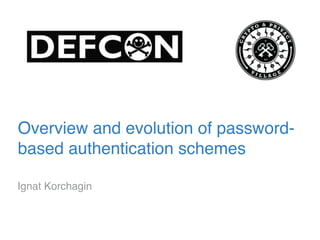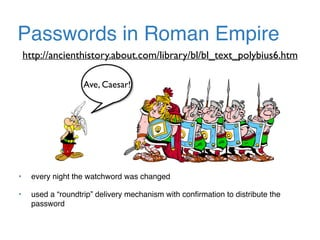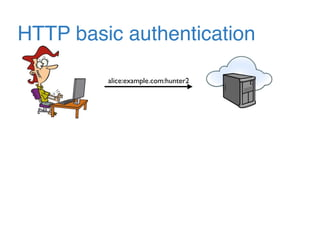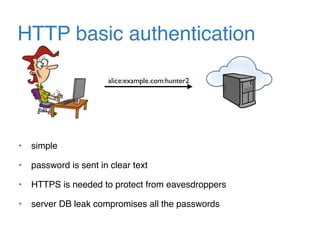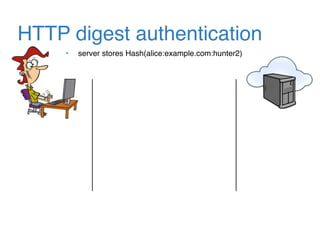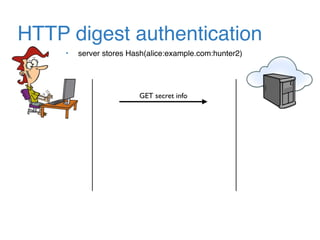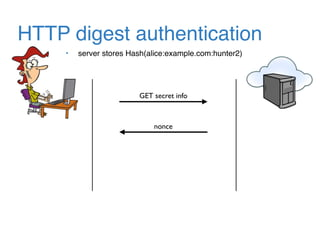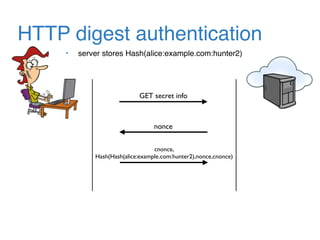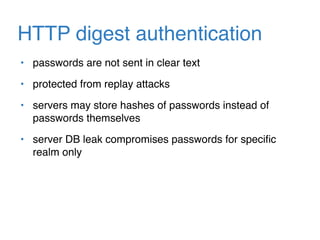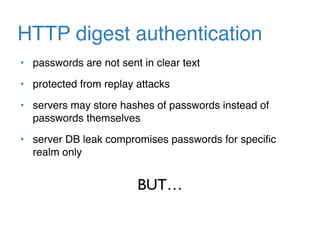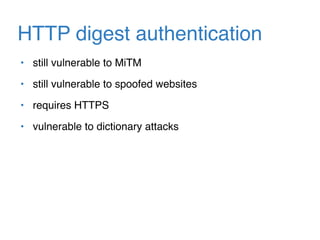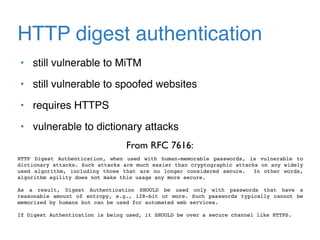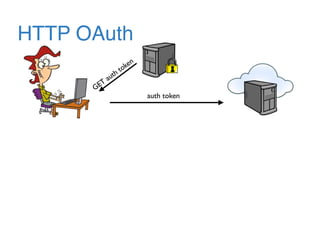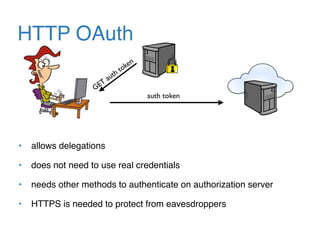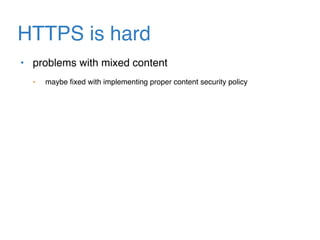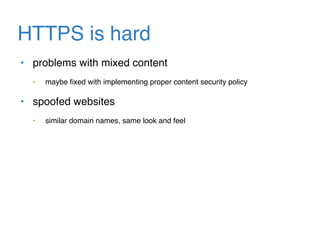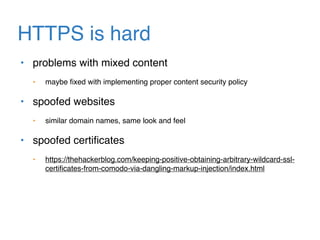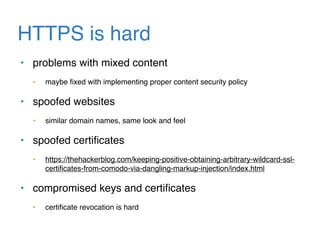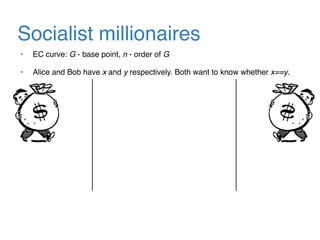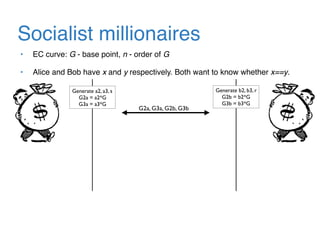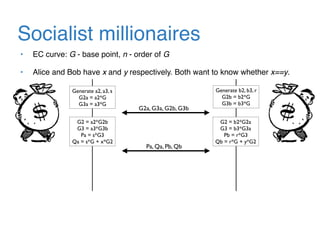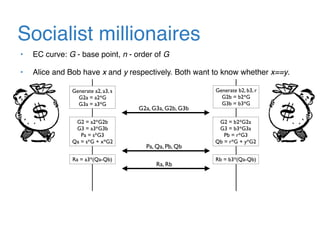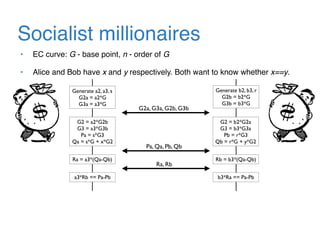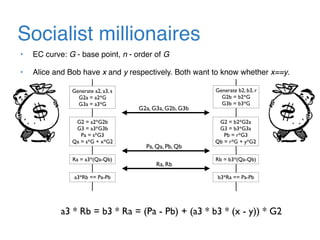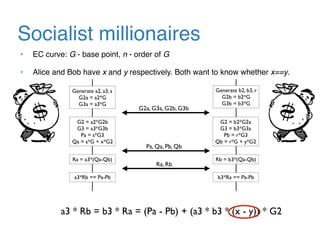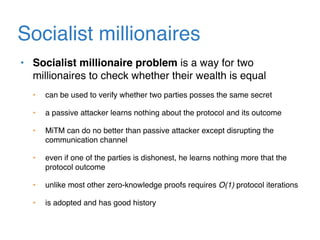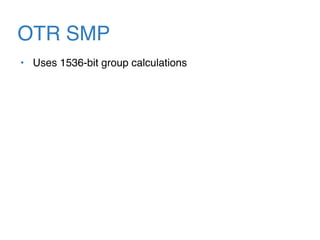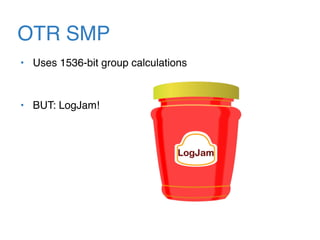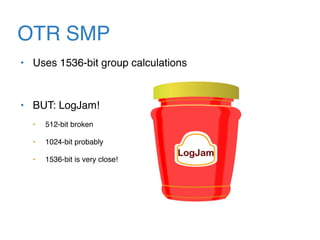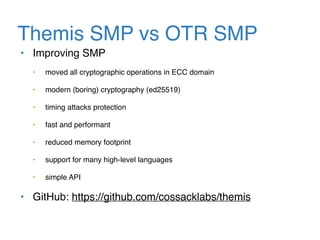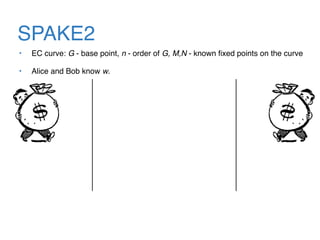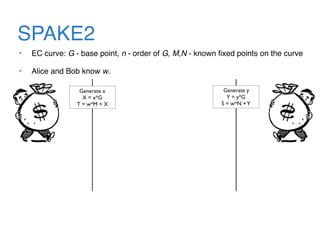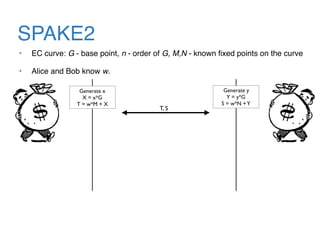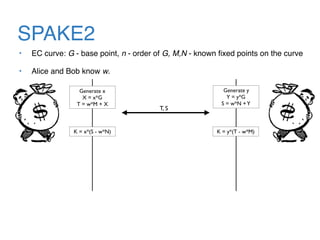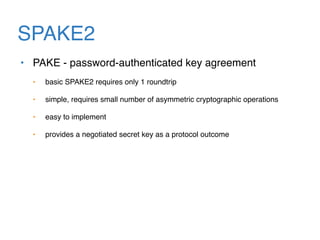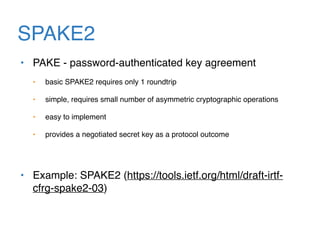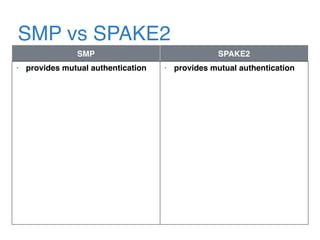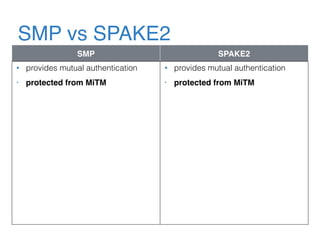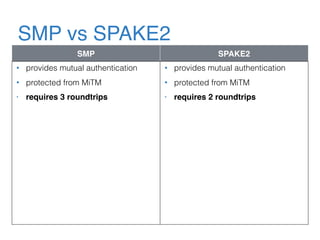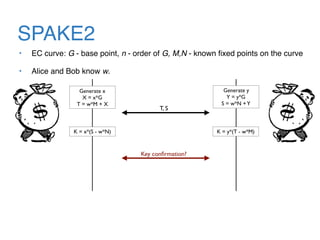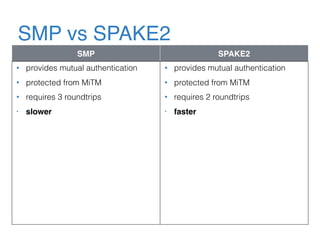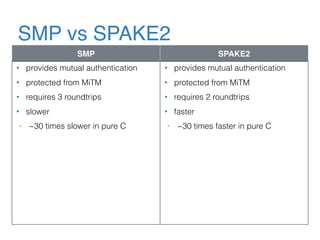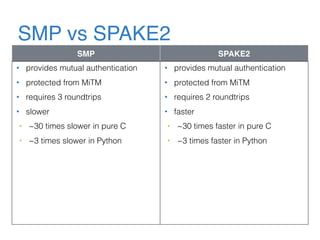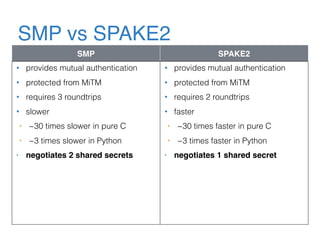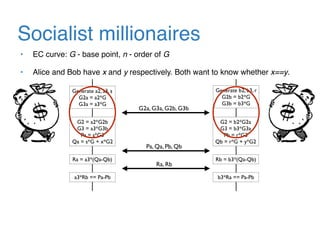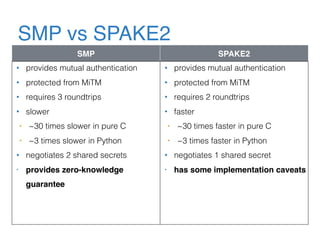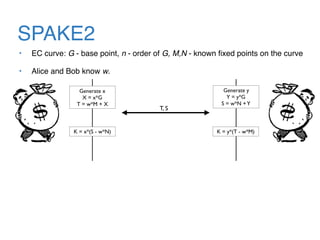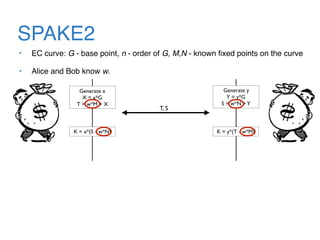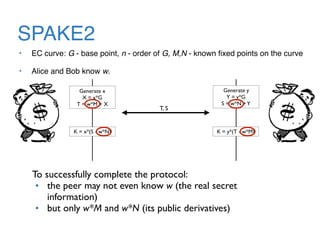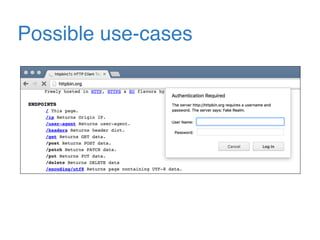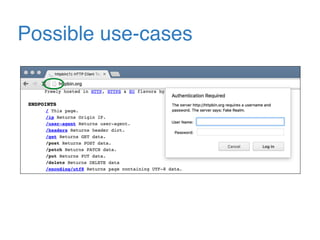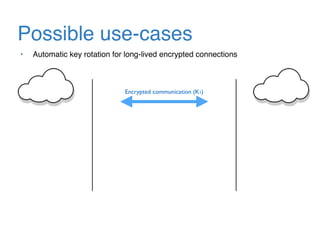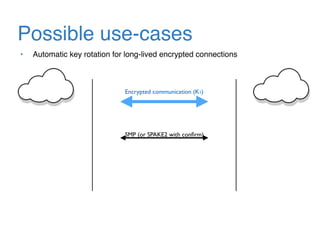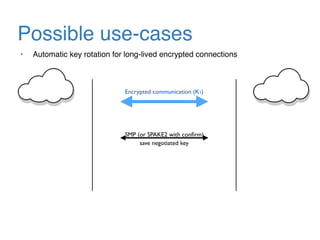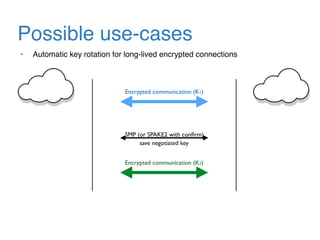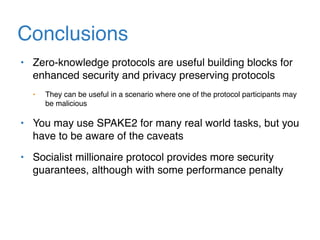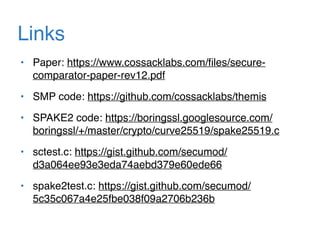1 of 65

### Overview and evolution of password-based authentication schemes

• 1. Overview and evolution of password- based authentication schemes Ignat Korchagin
• 2. Passwords in Roman Empire Ave, Caesar! http://ancienthistory.about.com/library/bl/bl_text_polybius6.htm • every night the watchword was changed • used a “roundtrip” delivery mechanism with conﬁrmation to distribute the password
• 3. Passwords in modern world create password? “hunter2” hehe, no one will ever guess
• 4. HTTP basic authentication alice:example.com:hunter2
• 5. HTTP basic authentication alice:example.com:hunter2 • simple • password is sent in clear text • HTTPS is needed to protect from eavesdroppers • server DB leak compromises all the passwords
• 6. HTTP digest authentication • server stores Hash(alice:example.com:hunter2)
• 7. HTTP digest authentication • server stores Hash(alice:example.com:hunter2) GET secret info
• 8. HTTP digest authentication • server stores Hash(alice:example.com:hunter2) GET secret info nonce
• 9. HTTP digest authentication • server stores Hash(alice:example.com:hunter2) GET secret info nonce cnonce, Hash(Hash(alice:example.com:hunter2),nonce,cnonce)
• 10. HTTP digest authentication • passwords are not sent in clear text • protected from replay attacks • servers may store hashes of passwords instead of passwords themselves • server DB leak compromises passwords for speciﬁc realm only
• 11. HTTP digest authentication • passwords are not sent in clear text • protected from replay attacks • servers may store hashes of passwords instead of passwords themselves • server DB leak compromises passwords for speciﬁc realm only BUT…
• 12. HTTP digest authentication • still vulnerable to MiTM • still vulnerable to spoofed websites • requires HTTPS • vulnerable to dictionary attacks
• 13. HTTP digest authentication • still vulnerable to MiTM • still vulnerable to spoofed websites • requires HTTPS • vulnerable to dictionary attacks From RFC 7616: HTTP Digest Authentication, when used with human-memorable passwords, is vulnerable to dictionary attacks. Such attacks are much easier than cryptographic attacks on any widely used algorithm, including those that are no longer considered secure. In other words, algorithm agility does not make this usage any more secure. As a result, Digest Authentication SHOULD be used only with passwords that have a reasonable amount of entropy, e.g., 128-bit or more. Such passwords typically cannot be memorized by humans but can be used for automated web services. If Digest Authentication is being used, it SHOULD be over a secure channel like HTTPS.
• 14. HTTP OAuth auth token GET auth token
• 15. HTTP OAuth auth token GET auth token • allows delegations • does not need to use real credentials • needs other methods to authenticate on authorization server • HTTPS is needed to protect from eavesdroppers
• 16. HTTPS is hard
• 17. HTTPS is hard • problems with mixed content • maybe ﬁxed with implementing proper content security policy
• 18. HTTPS is hard • problems with mixed content • maybe ﬁxed with implementing proper content security policy • spoofed websites • similar domain names, same look and feel
• 19. HTTPS is hard • problems with mixed content • maybe ﬁxed with implementing proper content security policy • spoofed websites • similar domain names, same look and feel • spoofed certiﬁcates • https://thehackerblog.com/keeping-positive-obtaining-arbitrary-wildcard-ssl- certiﬁcates-from-comodo-via-dangling-markup-injection/index.html
• 20. HTTPS is hard • problems with mixed content • maybe ﬁxed with implementing proper content security policy • spoofed websites • similar domain names, same look and feel • spoofed certiﬁcates • https://thehackerblog.com/keeping-positive-obtaining-arbitrary-wildcard-ssl- certiﬁcates-from-comodo-via-dangling-markup-injection/index.html • compromised keys and certiﬁcates • certiﬁcate revocation is hard
• 21. Can we do better?
• 22. Socialist millionaires • Socialist millionaire problem is a way for two millionaires to check whether their wealth is equal
• 23. Socialist millionaires • EC curve: G - base point, n - order of G • Alice and Bob have x and y respectively. Both want to know whether x==y.
• 24. Socialist millionaires • EC curve: G - base point, n - order of G • Alice and Bob have x and y respectively. Both want to know whether x==y. Generate a2, a3, s G2a = a2*G G3a = a3*G Generate b2, b3, r G2b = b2*G G3b = b3*G G2a, G3a, G2b, G3b
• 25. Socialist millionaires • EC curve: G - base point, n - order of G • Alice and Bob have x and y respectively. Both want to know whether x==y. Generate a2, a3, s G2a = a2*G G3a = a3*G Generate b2, b3, r G2b = b2*G G3b = b3*G G2 = a2*G2b G3 = a3*G3b Pa = s*G3 Qa = s*G + x*G2 G2 = b2*G2a G3 = b3*G3a Pb = r*G3 Qb = r*G + y*G2 G2a, G3a, G2b, G3b Pa, Qa, Pb, Qb
• 26. Socialist millionaires • EC curve: G - base point, n - order of G • Alice and Bob have x and y respectively. Both want to know whether x==y. Generate a2, a3, s G2a = a2*G G3a = a3*G Generate b2, b3, r G2b = b2*G G3b = b3*G G2 = a2*G2b G3 = a3*G3b Pa = s*G3 Qa = s*G + x*G2 G2 = b2*G2a G3 = b3*G3a Pb = r*G3 Qb = r*G + y*G2 Ra = a3*(Qa-Qb) Rb = b3*(Qa-Qb) G2a, G3a, G2b, G3b Pa, Qa, Pb, Qb Ra, Rb
• 27. Socialist millionaires • EC curve: G - base point, n - order of G • Alice and Bob have x and y respectively. Both want to know whether x==y. Generate a2, a3, s G2a = a2*G G3a = a3*G Generate b2, b3, r G2b = b2*G G3b = b3*G G2 = a2*G2b G3 = a3*G3b Pa = s*G3 Qa = s*G + x*G2 G2 = b2*G2a G3 = b3*G3a Pb = r*G3 Qb = r*G + y*G2 Ra = a3*(Qa-Qb) Rb = b3*(Qa-Qb) a3*Rb == Pa-Pb b3*Ra == Pa-Pb G2a, G3a, G2b, G3b Pa, Qa, Pb, Qb Ra, Rb
• 28. Socialist millionaires • EC curve: G - base point, n - order of G • Alice and Bob have x and y respectively. Both want to know whether x==y. Generate a2, a3, s G2a = a2*G G3a = a3*G Generate b2, b3, r G2b = b2*G G3b = b3*G G2 = a2*G2b G3 = a3*G3b Pa = s*G3 Qa = s*G + x*G2 G2 = b2*G2a G3 = b3*G3a Pb = r*G3 Qb = r*G + y*G2 Ra = a3*(Qa-Qb) Rb = b3*(Qa-Qb) a3*Rb == Pa-Pb b3*Ra == Pa-Pb G2a, G3a, G2b, G3b Pa, Qa, Pb, Qb Ra, Rb a3 * Rb = b3 * Ra = (Pa - Pb) + (a3 * b3 * (x - y)) * G2
• 29. Socialist millionaires • EC curve: G - base point, n - order of G • Alice and Bob have x and y respectively. Both want to know whether x==y. Generate a2, a3, s G2a = a2*G G3a = a3*G Generate b2, b3, r G2b = b2*G G3b = b3*G G2 = a2*G2b G3 = a3*G3b Pa = s*G3 Qa = s*G + x*G2 G2 = b2*G2a G3 = b3*G3a Pb = r*G3 Qb = r*G + y*G2 Ra = a3*(Qa-Qb) Rb = b3*(Qa-Qb) a3*Rb == Pa-Pb b3*Ra == Pa-Pb G2a, G3a, G2b, G3b Pa, Qa, Pb, Qb Ra, Rb a3 * Rb = b3 * Ra = (Pa - Pb) + (a3 * b3 * (x - y)) * G2
• 30. Socialist millionaires • Socialist millionaire problem is a way for two millionaires to check whether their wealth is equal • can be used to verify whether two parties posses the same secret • a passive attacker learns nothing about the protocol and its outcome • MiTM can do no better than passive attacker except disrupting the communication channel • even if one of the parties is dishonest, he learns nothing more that the protocol outcome • unlike most other zero-knowledge proofs requires O(1) protocol iterations • is adopted and has good history
• 31. OTR SMP • Uses 1536-bit group calculations
• 32. OTR SMP • Uses 1536-bit group calculations • BUT: LogJam!
• 33. OTR SMP • Uses 1536-bit group calculations • BUT: LogJam! • 512-bit broken • 1024-bit probably • 1536-bit is very close!
• 34. Themis SMP vs OTR SMP • Improving SMP • moved all cryptographic operations in ECC domain • modern (boring) cryptography (ed25519) • timing attacks protection • fast and performant • reduced memory footprint • support for many high-level languages • simple API • GitHub: https://github.com/cossacklabs/themis
• 35. SPAKE2 • EC curve: G - base point, n - order of G, M,N - known ﬁxed points on the curve • Alice and Bob know w.
• 36. SPAKE2 • EC curve: G - base point, n - order of G, M,N - known ﬁxed points on the curve • Alice and Bob know w. Generate x X = x*G T = w*M + X Generate y Y = y*G S = w*N +Y
• 37. SPAKE2 • EC curve: G - base point, n - order of G, M,N - known ﬁxed points on the curve • Alice and Bob know w. Generate x X = x*G T = w*M + X Generate y Y = y*G S = w*N +Y T, S
• 38. SPAKE2 • EC curve: G - base point, n - order of G, M,N - known ﬁxed points on the curve • Alice and Bob know w. Generate x X = x*G T = w*M + X Generate y Y = y*G S = w*N +Y K = x*(S - w*N) K = y*(T - w*M) T, S
• 39. SPAKE2 • PAKE - password-authenticated key agreement • basic SPAKE2 requires only 1 roundtrip • simple, requires small number of asymmetric cryptographic operations • easy to implement • provides a negotiated secret key as a protocol outcome
• 40. SPAKE2 • PAKE - password-authenticated key agreement • basic SPAKE2 requires only 1 roundtrip • simple, requires small number of asymmetric cryptographic operations • easy to implement • provides a negotiated secret key as a protocol outcome • Example: SPAKE2 (https://tools.ietf.org/html/draft-irtf- cfrg-spake2-03)
• 41. SMP vs SPAKE2 SMP SPAKE2 • provides mutual authentication • provides mutual authentication
• 42. SMP vs SPAKE2 SMP SPAKE2 • provides mutual authentication • protected from MiTM • provides mutual authentication • protected from MiTM
• 43. SMP vs SPAKE2 SMP SPAKE2 • provides mutual authentication • protected from MiTM • requires 3 roundtrips • provides mutual authentication • protected from MiTM • requires 2 roundtrips
• 44. Socialist millionaires • EC curve: G - base point, n - order of G • Alice and Bob have x and y respectively. Both want to know whether x==y. Generate a2, a3, s G2a = a2*G G3a = a3*G Generate b2, b3, r G2b = b2*G G3b = b3*G G2 = a2*G2b G3 = a3*G3b Pa = s*G3 Qa = s*G + x*G2 G2 = b2*G2a G3 = b3*G3a Pb = r*G3 Qb = r*G + y*G2 Ra = a3*(Qa-Qb) Rb = b3*(Qa-Qb) a3*Rb == Pa-Pb b3*Ra == Pa-Pb G2a, G3a, G2b, G3b Pa, Qa, Pb, Qb Ra, Rb
• 45. SPAKE2 • EC curve: G - base point, n - order of G, M,N - known ﬁxed points on the curve • Alice and Bob know w. Generate x X = x*G T = w*M + X Generate y Y = y*G S = w*N +Y K = x*(S - w*N) K = y*(T - w*M) T, S
• 46. SPAKE2 • EC curve: G - base point, n - order of G, M,N - known ﬁxed points on the curve • Alice and Bob know w. Generate x X = x*G T = w*M + X Generate y Y = y*G S = w*N +Y K = x*(S - w*N) K = y*(T - w*M) T, S Key conﬁrmation?
• 47. SMP vs SPAKE2 SMP SPAKE2 • provides mutual authentication • protected from MiTM • requires 3 roundtrips • slower • provides mutual authentication • protected from MiTM • requires 2 roundtrips • faster
• 48. SMP vs SPAKE2 SMP SPAKE2 • provides mutual authentication • protected from MiTM • requires 3 roundtrips • slower • ~30 times slower in pure C • provides mutual authentication • protected from MiTM • requires 2 roundtrips • faster • ~30 times faster in pure C
• 49. SMP vs SPAKE2 SMP SPAKE2 • provides mutual authentication • protected from MiTM • requires 3 roundtrips • slower • ~30 times slower in pure C • ~3 times slower in Python • provides mutual authentication • protected from MiTM • requires 2 roundtrips • faster • ~30 times faster in pure C • ~3 times faster in Python
• 50. SMP vs SPAKE2 SMP SPAKE2 • provides mutual authentication • protected from MiTM • requires 3 roundtrips • slower • ~30 times slower in pure C • ~3 times slower in Python • negotiates 2 shared secrets • provides mutual authentication • protected from MiTM • requires 2 roundtrips • faster • ~30 times faster in pure C • ~3 times faster in Python • negotiates 1 shared secret
• 51. Socialist millionaires • EC curve: G - base point, n - order of G • Alice and Bob have x and y respectively. Both want to know whether x==y. Generate a2, a3, s G2a = a2*G G3a = a3*G Generate b2, b3, r G2b = b2*G G3b = b3*G G2 = a2*G2b G3 = a3*G3b Pa = s*G3 Qa = s*G + x*G2 G2 = b2*G2a G3 = b3*G3a Pb = r*G3 Qb = r*G + y*G2 Ra = a3*(Qa-Qb) Rb = b3*(Qa-Qb) a3*Rb == Pa-Pb b3*Ra == Pa-Pb G2a, G3a, G2b, G3b Pa, Qa, Pb, Qb Ra, Rb
• 52. Socialist millionaires • EC curve: G - base point, n - order of G • Alice and Bob have x and y respectively. Both want to know whether x==y. Generate a2, a3, s G2a = a2*G G3a = a3*G Generate b2, b3, r G2b = b2*G G3b = b3*G G2 = a2*G2b G3 = a3*G3b Pa = s*G3 Qa = s*G + x*G2 G2 = b2*G2a G3 = b3*G3a Pb = r*G3 Qb = r*G + y*G2 Ra = a3*(Qa-Qb) Rb = b3*(Qa-Qb) a3*Rb == Pa-Pb b3*Ra == Pa-Pb G2a, G3a, G2b, G3b Pa, Qa, Pb, Qb Ra, Rb
• 53. SMP vs SPAKE2 SMP SPAKE2 • provides mutual authentication • protected from MiTM • requires 3 roundtrips • slower • ~30 times slower in pure C • ~3 times slower in Python • negotiates 2 shared secrets • provides zero-knowledge guarantee • provides mutual authentication • protected from MiTM • requires 2 roundtrips • faster • ~30 times faster in pure C • ~3 times faster in Python • negotiates 1 shared secret • has some implementation caveats
• 54. SPAKE2 • EC curve: G - base point, n - order of G, M,N - known ﬁxed points on the curve • Alice and Bob know w. Generate x X = x*G T = w*M + X Generate y Y = y*G S = w*N +Y K = x*(S - w*N) K = y*(T - w*M) T, S
• 55. SPAKE2 • EC curve: G - base point, n - order of G, M,N - known ﬁxed points on the curve • Alice and Bob know w. Generate x X = x*G T = w*M + X Generate y Y = y*G S = w*N +Y K = x*(S - w*N) K = y*(T - w*M) T, S
• 56. SPAKE2 • EC curve: G - base point, n - order of G, M,N - known ﬁxed points on the curve • Alice and Bob know w. Generate x X = x*G T = w*M + X Generate y Y = y*G S = w*N +Y K = x*(S - w*N) K = y*(T - w*M) T, S To successfully complete the protocol: • the peer may not even know w (the real secret information) • but only w*M and w*N (its public derivatives)
• 57. Possible use-cases
• 58. Possible use-cases
• 59. Possible use-cases Encrypted communication (K1) • Automatic key rotation for long-lived encrypted connections
• 60. Possible use-cases SMP (or SPAKE2 with conﬁrm) Encrypted communication (K1) • Automatic key rotation for long-lived encrypted connections
• 61. Possible use-cases SMP (or SPAKE2 with conﬁrm) Encrypted communication (K1) • Automatic key rotation for long-lived encrypted connections save negotiated key
• 62. Possible use-cases SMP (or SPAKE2 with conﬁrm) Encrypted communication (K1) Encrypted communication (K2) • Automatic key rotation for long-lived encrypted connections save negotiated key
• 63. Conclusions • Zero-knowledge protocols are useful building blocks for enhanced security and privacy preserving protocols • They can be useful in a scenario where one of the protocol participants may be malicious • You may use SPAKE2 for many real world tasks, but you have to be aware of the caveats • Socialist millionaire protocol provides more security guarantees, although with some performance penalty
• 64. Links • Paper: https://www.cossacklabs.com/ﬁles/secure- comparator-paper-rev12.pdf • SMP code: https://github.com/cossacklabs/themis • SPAKE2 code: https://boringssl.googlesource.com/ boringssl/+/master/crypto/curve25519/spake25519.c • sctest.c: https://gist.github.com/secumod/ d3a064ee93e3eda74aebd379e60ede66 • spake2test.c: https://gist.github.com/secumod/ 5c35c067a4e25fbe038f09a2706b236b
• 65. Thank you! Questions?